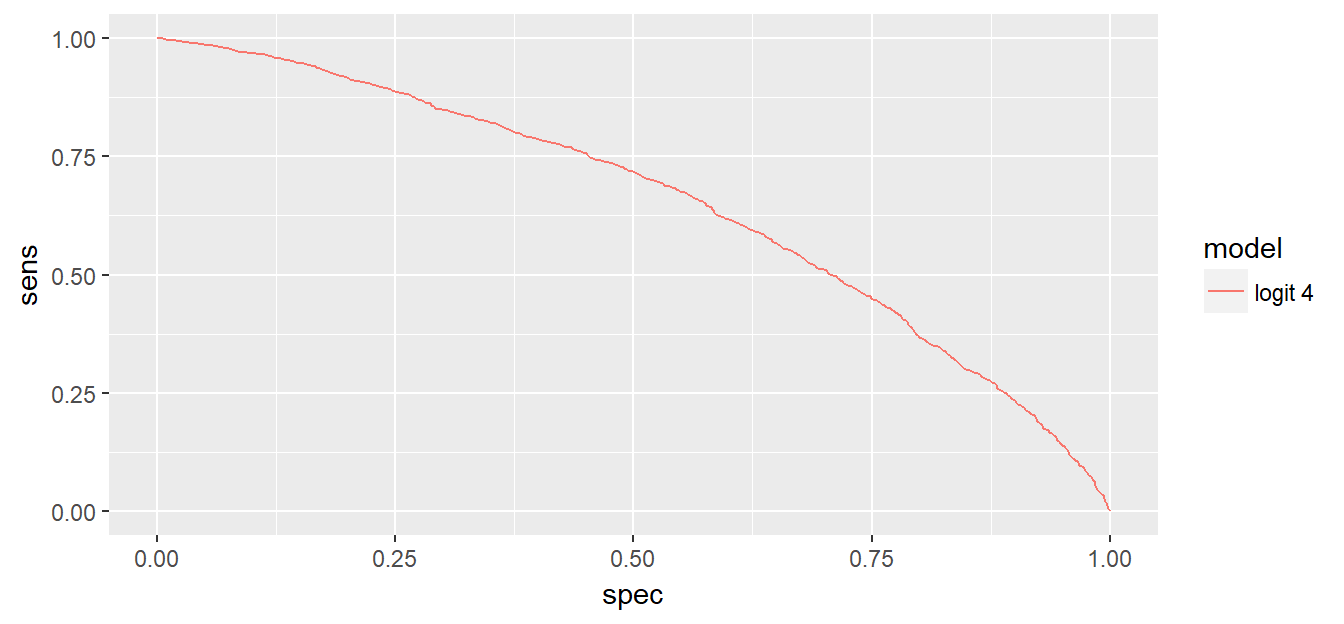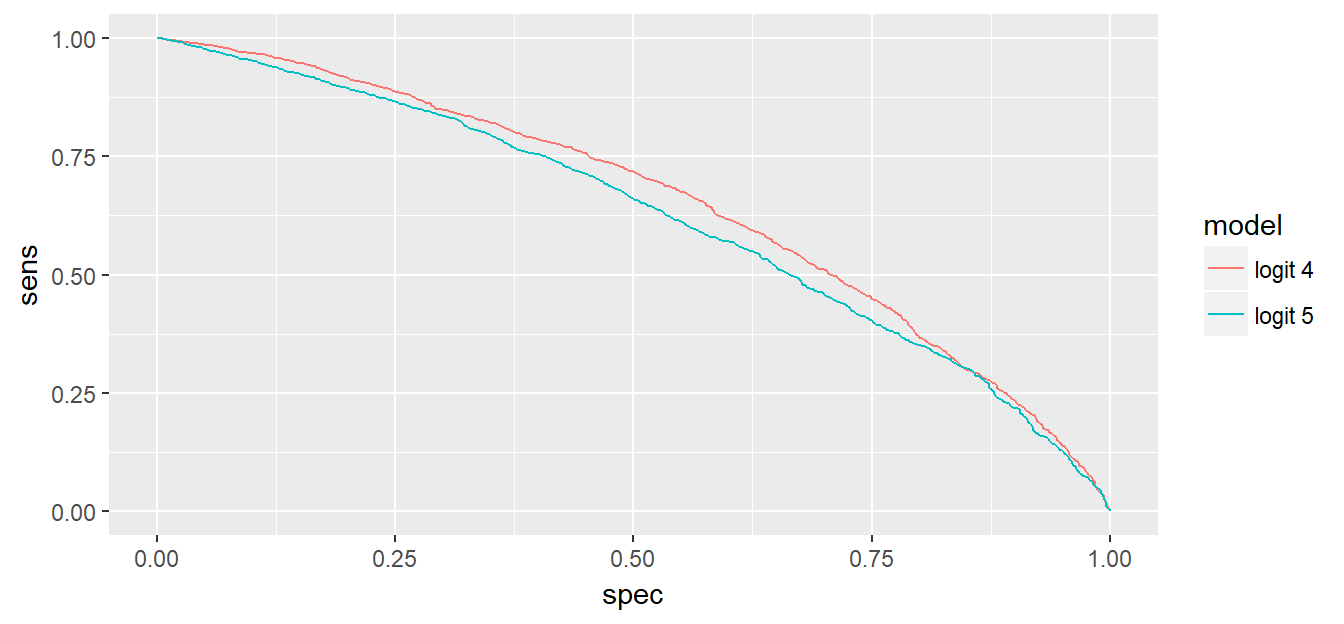# Lab 10: Trade-off between sensitivity and specificity

### Learning Objectives:

• predicting with logistic regression
• two types of errors, two types of correct classification (sensitivity and specificity)
• fundamental trade-off between sensitivity and specificity
• kappa and confusionMatrix()
• ROC curves

The background reading for this lab is chapter 10 in Lantz.

### 1. Predicting with a logistic model

In lab 9 we estimated a logistic model using all of the observations. This is appropriate in a “confirmatory” analysis where we test a particular hypothesis. In this class however, we are interested in making predictions - using past data to predict the future. In order to test how well our predictions might work on future data we always split our data into train and test. We then estimate our model on train data, and evaluate our predictions in the test data. Let’s use 80/20 train/test split.

library(tidyverse)
library(descr)
loan <- read_csv("lending_club_cleaned.csv")
loan$status <- as.factor(loan$status)
set.seed(364)
sample <- sample(nrow(loan),floor(nrow(loan)*0.8))
train <- loan[sample,]
test <- loan[-sample,]

Let’s estimate the model with fico, dti, loan_amnt and purpose as predictors. predict() function returns probabilities that loan is good.

logit4 <- glm(status ~ fico + dti+ loan_amnt + purpose, data = train, family = "binomial")
test$pred <- predict(logit4, test, type="response") head(test$pred)
##  0.9044742 0.8220173 0.8638981 0.8550825 0.8062179 0.8413071
summary(test$pred) ## Min. 1st Qu. Median Mean 3rd Qu. Max. ## 0.4347 0.8107 0.8563 0.8498 0.8979 0.9719 We can see that the estimated probabilities of a loan being good range from 0.43 to 0.97 in the test data set. What probability cutoff should we use for classifying a loan as “good”? For now, let’s classify any loan that has more than 0.8 probability of being good as “good”, the rest is classified as “bad”. test$status_pred80 <- ifelse(test$pred > 0.80, "good", "bad") crosstab(test$status,test$status_pred80, prop.t=TRUE, plot=FALSE) ## Cell Contents ## |-------------------------| ## | Count | ## | Total Percent | ## |-------------------------| ## ## ==================================== ## test$status_pred80
spec=roc$specificities, model="logit 4") ggplot(roc_curves, aes(x=spec, y=sens, color=model)) + geom_line()### 7. Using ROC curves to horse-race algorithms Let’s plot ROC curves for logit4 and logit5 models in the same graph. We will be able to see each algorithm’s performance in terms of sensitivity and specificity. logit5 <- glm(status ~ fico + dti, data = train, family = "binomial") test$pred2 <- predict(logit5, test, type="response")
roc <- roc(test$status,test$pred2)
roc_curve2 <- data.frame(sens=roc$sensitivities, spec=roc$specificities,
model="logit 5")
roc_curves <- bind_rows(roc_curves, roc_curve2)
ggplot(roc_curves, aes(x=spec, y=sens, color=model)) + geom_line()Clearly, logit4 algorithm performs better as it has higher sensitivity and specificity across all of the ranges. This is not surprising give that logit4 has all of the variables including in logit5.

### Exercises

1. Let’s load the NHL data with cumulative wins information (nhl with cum wins.csv). Set seed to 364. Create train data set using games before March 15, 2016, and test data set would include data/games after March 15. Thus, we will try to predict a lot more games than we did in the past, 194 to be precise.

2. Estimate a logit model using cumwins_net as the only explanatory variable. Is the effect of cumwins_net on the odds of home team winning statistically significant? What is the magnitude of the effect?

3. Calculate the probability of the home team winning for every game after and including March 15, i.e. all the games in the test data set. The outcome of which two games are you most confident predicting?

4. Make predictions for the outcomes (homewin) of the games in the test data. Use 0.5 as the cutoff probability for home team winning or losing. Are you happy with the performance of these predictions? What is your Kappa?

5. Can you change the probability cutoff and improve on your predictions? Explain your answer.

6. If positive class is that home team loses, what is your sensitivity? What probability cutoff would you choose to make sensitivity really high? What will happen to your specificity?

7. Plot the ROC curve for your logit model with cumwins_net as the only predictor.

8. Estimate an alternative logit model with cumwins_home and cumwins_visit as the only predictors. Plot the ROC curve for this and the model from question 7. Which model do you prefer, if any?